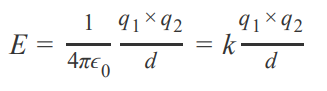# Problem: The overall energy involved in the formation of CsCl from Cs(s) and Cl2 (g) is − 443 kJ/mol. Given the following information:heat of sublimation for Cs is +76 kJ/molbond dissociation energy for 1/2 Cl2 is +121 kJ/mol,Ei1 for Cs is +376 kJ/mol, andEea for Cl(g) is − 349 kJ/mol.what is the magnitude of the lattice energy for CsCl?The electrostatic attraction between oppositely charged ions is called an ionic bond. The net energy change in the formation of an ionic bond is a composite of five steps, some of which absorb energy and some of which release energy. The steps are summarized for a generic metal, M, and a diatomic halogen, X2 , as1. sublimation of the metal2. dissociation of the X−X bond,3. ionization of the metal,4. transfer of the electron to X, and5. crystalline solid formation.The overall energy transfer is the sum of the energy changes for each step. The magnitude of the potential energy of two interacting charges, EE, (which is related to Lattice energy) is the energy associated with the strength of the attraction between ions in the ionic compound and can be determined bywhere k is a constant equal to 1/(4πϵ0) = 1/(1.11 × 10 − 10 C2 /(J ⋅ m)), q1 and q2 are the charges of the ions, and dd is the distance between the nuclei of the ions.

###### FREE Expert Solution
93% (486 ratings)View Complete Written Solution
###### Problem Details

The overall energy involved in the formation of CsCl from Cs(s) and Cl2 (g) is − 443 kJ/mol. Given the following information:

• heat of sublimation for Cs is +76 kJ/mol
• bond dissociation energy for 1/2 Cl2 is +121 kJ/mol,
• Ei1 for Cs is +376 kJ/mol, and
• Eea for Cl(g) is − 349 kJ/mol.

what is the magnitude of the lattice energy for CsCl?

The electrostatic attraction between oppositely charged ions is called an ionic bond. The net energy change in the formation of an ionic bond is a composite of five steps, some of which absorb energy and some of which release energy. The steps are summarized for a generic metal, M, and a diatomic halogen, X2 , as

1. sublimation of the metal
2. dissociation of the X−X bond,
3. ionization of the metal,
4. transfer of the electron to X, and
5. crystalline solid formation.

The overall energy transfer is the sum of the energy changes for each step. The magnitude of the potential energy of two interacting charges, EE, (which is related to Lattice energy) is the energy associated with the strength of the attraction between ions in the ionic compound and can be determined bywhere k is a constant equal to 1/(4πϵ0) = 1/(1.11 × 10 − 10 C2 /(J ⋅ m)), q1 and q2 are the charges of the ions, and dd is the distance between the nuclei of the ions.